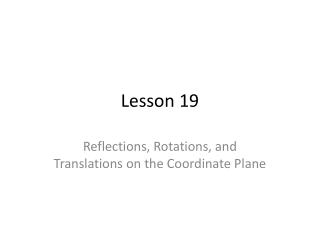DownloadDownload PresentationLesson 19

# Lesson 19

Download Presentation## Lesson 19

- - - - - - - - - - - - - - - - - - - - - - - - - - - E N D - - - - - - - - - - - - - - - - - - - - - - - - - - -
##### Presentation Transcript

1. Lesson 19 Reflections, Rotations, and Translations on the Coordinate Plane

2. Transformations • A transformation can be used to change a figures position. • The first type we will discuss is a reflection. This is a flip of a figure across a point of an axis. The result will look like a mirror image of the pre-image. (the pre-image is the figure you start off with, before you perform a transformation).

3. Of course, there are rules • If a point is reflected over the x –axis, every point (x,y) becomes (x,-y). • If a point is reflected over the y-axis, every point (x,y) becomes (-x, y).

4. Example 1 • Reflect Triangle ABC across the x-axis. • Pt A (2,2); pt B (4,6); pt c (8,3) • New points (2, -2) (4, -6) (8,-3)

5. Rotation • The second type of transformation is rotation. A rotation is a turn of a figure around a point. • 90° counter clockwise 180 °

6. Example 2 • Rotate Triangle PQR 180 clockwise around point Q. { pt P (1,1); pt Q (4,1); pt R (4,3)} • Since we are rotating around pt Q, it stays in the same spot. • What happens to pt P? • After 180° rotation, point P will be at (7,1) • What about pt R? • Point R will be at (4, -1)

7. What about a rotation around the origin? • What if we were asked to rotate an object around the origin (0,0)? There is a short cut we can use!! • If you are asked to rotate 90 clockwise: your point (x,y) becomes (y,-x). • If you are asked to rotate 90counter clockwise: your point (x,y) becomes (-y, x) • If you are asked to rotate 180clockwise OR counter clockwise, point (x,y) becomes (-x,-y)

8. Example 2a • Rotate Triangle PQR 90 clockwise around the origin. { pt P (1,1); pt Q (4,1); pt R (4,3)} • Since we are rotating around the origin, the shape is going to move from ne quadrant to the next. • Point P will become (1,-1) • Point Q will become (1,-4) • Point R will become (3, -4) R Q P P R Q

9. Translation • A translation is a slide of a figure to another location on a coordinate plane. • When a figure it translated, your figure is moved along the x axis (right and left) and/or along the y axis (up and down). • Every point (x,y) becomes (x+a, y+b). • A is adding your right or left movement to x. • B is adding your up or down movement to y.

10. Example 3 • Triangle HJK is translated 6 units to the left. Identify its coordinates. • H (2,2); K (6,3); J (4,4) • What would 6 units left look like with numbers? Moving left will change x. • H (2-6,2); K (6-6,3); J (4-6,4) • The new coordinates are: H1(-4,2); K1 (0,3); J1 (-2,4)

11. Let’s try some practice problems • Take out you Coach book and lets put what we learned to practice. • Complete numbers 1-6 on pages 122-123. We will complete the open ended together so we can review the SEA method. • Remember, if you are stuck, consult a partner.